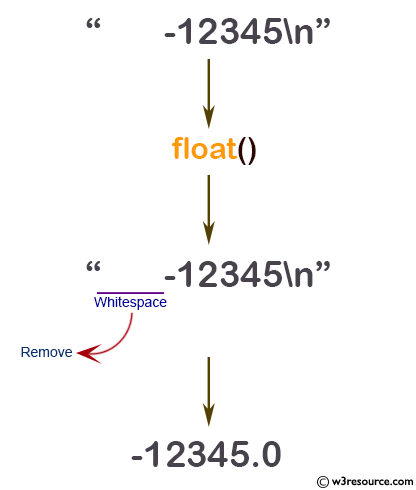# Python: float() function

## float() function

The float() function is used to convert the specified value into a floating point number.

Note: If the argument is a string, it should contain a decimal number, optionally preceded by a sign, and optionally embedded in whitespace. The optional sign may be '+' or '-'; a '+' sign has no effect on the value produced. The argument may also be a string representing a NaN (not-a-number), or a positive or negative infinity. The following grammar after leading and trailing whitespace characters are removed:

```sign           ::=  "+" | "-"
infinity       ::=  "Infinity" | "inf"
nan            ::=  "nan"
numeric_value  ::=  floatnumber | infinity | nan
numeric_string ::=  [sign] numeric_value
```

Return value :

If no argument is given, 0.0 is returned.

Version

(Python 3.2.5)

Syntax:

```float([x])
```

Return value:

Floating point number, if no argument is given, 0.0 is returned.

Example: Python float() function

``````# for floats
print(float(2.25))
# for string floats with whitespaces
print(float("       -12345\n"))
# for string floats
print(float("-15.55"))
print(float("1e-005"))
print(float("+1E7"))
print(float("-Infinity"))
``````

Output:

```2.25
-12345.0
-15.55
1e-05
10000000.0
-inf
```

Pictorial Presentation:The float type is described in Numeric Types — int, float, complex.

Python Code Editor:

Previous: filter()
Next: format()

Test your Python skills with w3resource's quiz

﻿

## Python: Tips of the Day

How do I check if a list is empty?

For example, if passed the following:

```a = []
if not a:
print("List is empty")
```

Ref: https://bit.ly/2A4JXx9